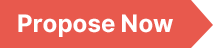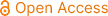### International Journal of Applied Mathematics and Theoretical Physics

Archive
• 2023, Volume 9

• 2022, Volume 8

• 2021, Volume 7

• 2020, Volume 6

• 2019, Volume 5

• 2018, Volume 4

• 2017, Volume 3

• 2016, Volume 2

• 2015, Volume 1

Submit a Manuscript

Publishing with us to make your research visible to the widest possible audience.Propose a Special Issue

Building a community of authors and readers to discuss the latest research and develop new ideas.### Comparison of the Coupled Solution of the Species, Mass, Momentum, and Energy Conservation Equations by Unstructured FVM, FDM, and FEM

In order to simulate fluid flow, heat transfer, and other related physical phenomena, it is necessary to describe the associated physics in mathematical terms. Nearly all the physical phenomena of interest to us in this book are governed by principles of conservation and are expressed in terms of partial differential equations expressing these principles. In this research paper, is a summary of conservation equations (Continuity, Momentum, Species, and Energy) that govern the flow of a Newtonian fluid. In particular, this paper studied the solution of two-dimensional (2D) Navier-Stokes (N-S) equations using the finite difference method (FDM), finite element method (FEM), and finite volume method (FVM) on a test problem of Methane combustion in a laminar diffusion flame. First, the computational domain was decomposed into grids in FDM and elements in FEM, later the Navier-Stokes equations, Energy, and, Species conservation equations were solved at the grid points and a MATLAB code has been written to check the consistency, stability, and accuracy for finer meshes. Following this step, the discretized equations for each sub-domain will be developed using the finite difference and finite element method, resolved using an iterative solver-Gauss Seidel technique. The MATLAB code is written for 2D geometries for science and engineering applications. The focus of this research paper is the development of physical models using numerical methods like FDM, and FEM for modeling science and Engineering applications by using Navier-stokes equations, Energy equations, and Species conservation equations.

Coupled Solution, Conservation Equations, FDM, FEM, FVM

Desta Sodano Sheiso, Saket Apparao Kuchibhotla. (2022). Comparison of the Coupled Solution of the Species, Mass, Momentum, and Energy Conservation Equations by Unstructured FVM, FDM, and FEM. International Journal of Applied Mathematics and Theoretical Physics, 8(3), 52-57. https://doi.org/10.11648/j.ijamtp.20220803.11